# 真实性渲染技术 Photo-realistic rendering

## 1. 真实性渲染技术的概念

### 1.1 基本概念

Photo-realistic rendering is some techniques that is intended to realistically insert virtual object in the real-time physical environment in AR applications, These techniques include methods for light source acquisition through image-based lighting or sampling,registering and estimationg the lighting conditions, and composition of global illumination1.

### 1.3 与Non-photorealistic rendering的关系

Three-dimensional NPR is the style that is most commonly seen in video games and movies. The output from this technique is almost always a 3D model that has been modified from the original input model to portray a new artistic style.

The input to a two dimensional NPR system is typically an image or video. The output is a typically an artistic rendering of that input imagery (for example in a watercolor, painterly or sketched style) although some 2D NPR serves non-artistic purposes e.g. data visualization.

Artistic rendering3 is the application of visual art styles to rendering

## 2. 辐射基本概念与渲染方程

### 2.1 光学原理：辐射度量学相关概念

• 立体角

Radiant energy is the energy of electromannetic radiation. It is measured in units of joules, and denoted by the the symbol:
Q[J=Joule]

Randiant flux(power) is the energy emitted, reflected, transmitted or received, per unit time.
Φ = d Q d t , [ W = w a t t ] \varPhi=\frac{dQ}{dt},[W=watt]the radiant intensity is the power per unit solid angle emitted by a point light source.
I ( ω ) = d Φ d ω [ W s r ] I(\omega)=\frac{d\Phi}{d\omega}[\frac{W}{sr}]
the irradiance(flux density) is the radiant flux(power) per unit area incident on a suirface point
E = d Φ d A [ W m 2 ] E = \frac{d\Phi}{dA}[\frac{W}{m^2}]
辐照度即物体单位表面积接受的入射（incident）辐射通量。
the radiance (luminance) is the power emitted, reflected, transmitted or received by a surface, per unit solid angle, per unit area(perpendicular area).Or the irradiance per solid angle.
L = d E d ω = d 2 Φ d ω d A c o s θ L = \frac{dE}{d\omega}=\frac{d^2\Phi}{d\omega dAcos\theta}
辐射亮度是每单位立体角，每单位垂直面积的功率，它同时指定了光的方向与照射到的表面所接受到的亮度。
需要注意的是，辐射亮度强调的是单位垂直面积是指垂直于入射光线的照射面积。而 d A dA 是物体的表面积，因此存在一个入射角度 θ \theta ，因此需要乘 c o s θ cos\theta 转为垂直面积。通过对L进行积分，可以得到辐照度，### 2.2 双向反射分布方程（BRDF，bidirectional reflectence distribution function ）

• 光与表面的相互作用10
光通过物体表面时，可能会发生如下几种情况：
• 吸收
• 反射
• 镜面反射
• 漫反射
• 折射
• 定向折射
• 漫折射
• 散射
• 弹性散射
• 非弹性散射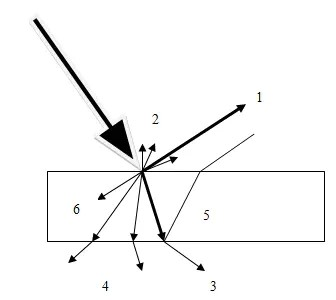1.镜面反射 2. 漫反射 3. 定向透射 4. 漫透射 5. 背反射 6. 吸收• 双向反射分布函数（Bidirectional reflectance distribution function, BRDF）
在不考虑散射的情况下，光从入射方向 Ψ \Psi 入射到表面的点 p p ，会通过漫反射和镜面反射从该点出反射到各个方面。BRDF就是来描述这一物理特性的函数。定义如下：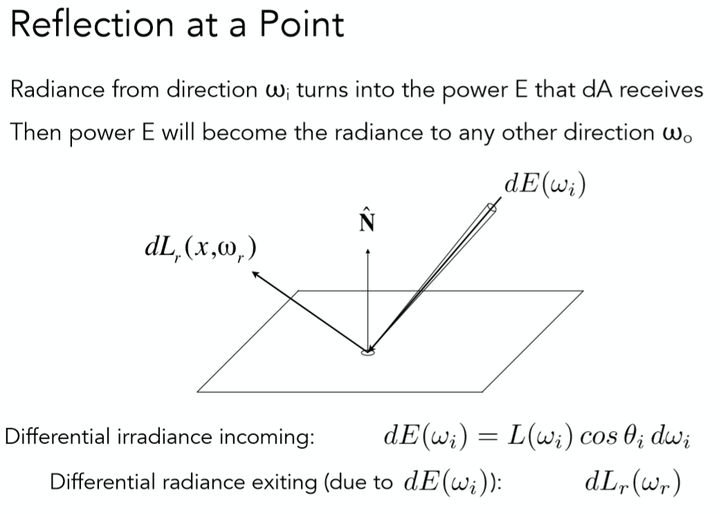一个点(微分面积元)在接受到一定方向上的亮度 d E ( ω i ) dE(\omega_i) 之后，再向不同方向把能量辐射出去( d L r ( ω r ) dL_r(\omega_r) )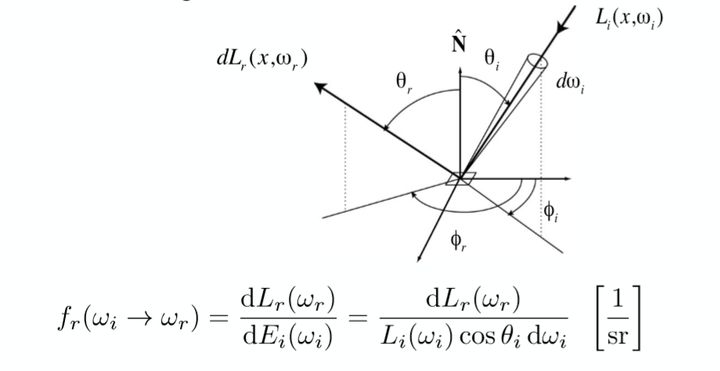• 反射方程
由BRDF，可以得到反射方程如下：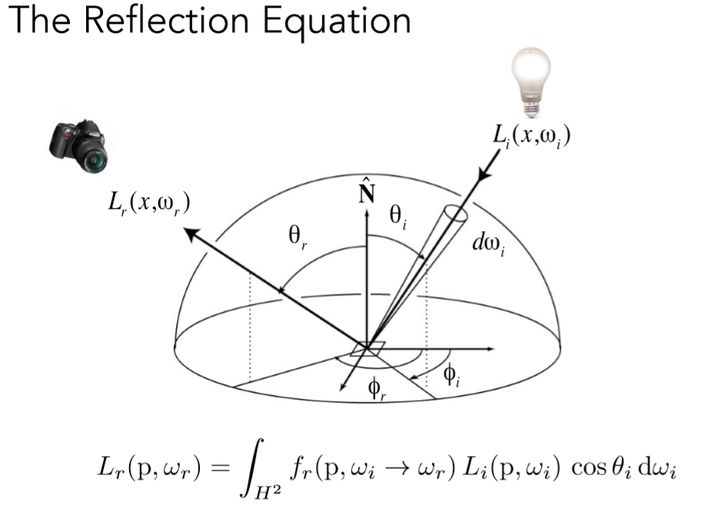即摄像机所接受到的[公式]方向上的反射光，是由所有不同方向上入射光线的irradiance贡献得到的(即图中式子的 L i ( p , ω i ) c o s θ i d ω i L_i(p,\omega_i)cos\theta_id\omega_i )，而不同方向入射光线的irradiance对反射方向KaTeX parse error: Undefined control sequence: \omgea at position 1: \̲o̲m̲g̲e̲a̲_r的贡献程度则由物体表面材质决定，所以乘上了一个BRDF函数。

### 2.3 渲染方程

L ( p , ω o ) = L e ( p , ω o ) + L r ( p , ω r ) = L e ( p , ω o ) + ∫ Ω + L i ( p , ω i ) f r ( p r , ω i , ω o ) ( n ω ˙ i ) d ω i L(p,\omega_o) = L_e(p,\omega_o)+L_r(p,\omega_r)=L_e(p,\omega_o)+\int_\Omega^+L_i(p,\omega_i)f_r(p_r,\omega_i,\omega_o)(n\dot\omega_i)d\omega_i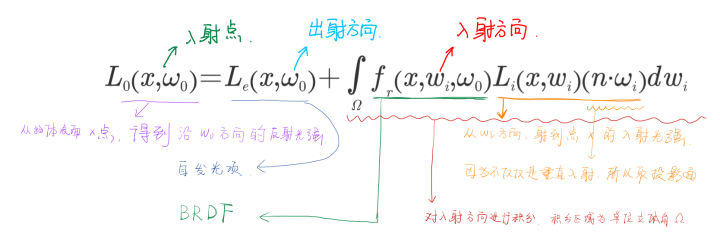L = E + K L L=E+KL

I L − K L = E ,   ( I 为 单 位 矩 阵 ) IL-KL = E, \ (I为单位矩阵)
( I − K ) L = E (I-K)L=E
L = ( I − K ) − 1 E L = (I-K)^{-1}E (尚未证明其可逆)

L = E + K E + K 2 E + K 3 E + . . . L=E+KE+K^2E+K^3E+...

### 2.4 球谐函数（SH，spherical harmonics）

1. 由广义二项式定理11: ( 1 − x ) − n = ∑ 0 ∞ C n r x r (1-x)^{-n}=\sum_0^{\infty}C_n^rx^r ,展开： ↩︎

2. 知乎：诺依曼级数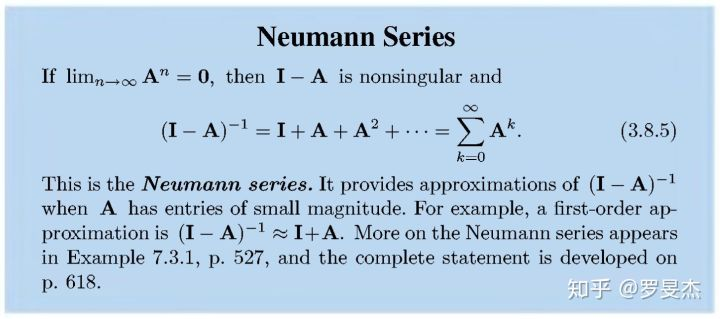↩︎

10-031万+
04-02818701-082232
02-258186
12-07165
11-191万+
08-08331
04-22849
08-104573
02-221223